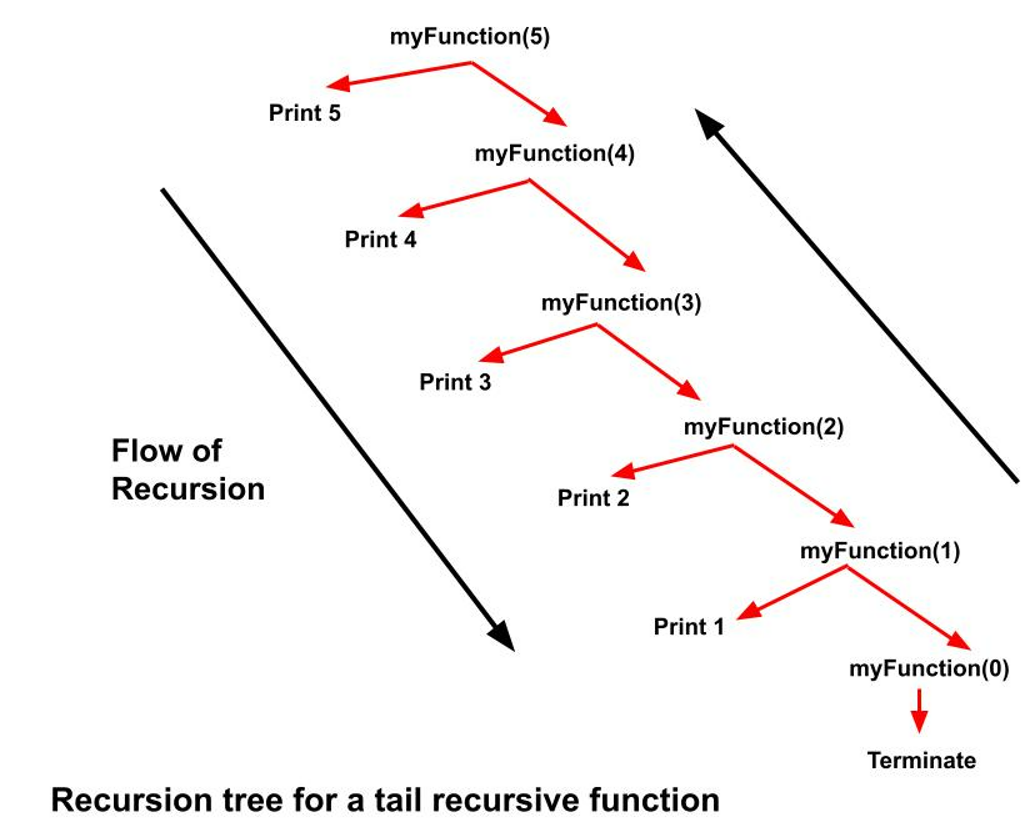# Tail Recursion : Explained

## What is a recursive function?

A function that continuously calls itself for a smaller input until it meets a breaking condition( base case of the recursion) is a recursive function.

Every recursive function must have a terminating condition(base case) to end the program otherwise it may fall into an infinite loop and since all recursive function calls are stored in the memory stack it may result in a stack overflow.

There are mainly 5 types of recursion:-

• Tail
• Tree
• Indirect
• Nested

## What is a Tail Recursion?

In a recursive function when the function call is made at the very end after executing all the statements above it then that function is a tail-recursive function.

Consider the following code to print numbers from N to 1:

Code:

## C++ Code

``````#include <bits/stdc++.h>
using namespace std;
void myFunction(int n) {
if (n > 0) {
cout << n << " ";
myFunction(n - 1);
}
}
int main() {
int n = 5;
myFunction(n);
return 0;
}
``````

Output: 5 4 3 2 1

Time Complexity: O(n)

Auxiliary Space:  O(1)

## Java Code

``````public class tUf {
static void myFunction(int n) {
if (n > 0) {
System.out.print(n + " ");
myFunction(n - 1);
}
}
public static void main(String args[]) {
int n = 5;
myFunction(n);
}
}
``````

Output: 5 4 3 2 1

Time Complexity: O(n)

Auxiliary Space:  O(1)

## Python Code

``````def myFunction(n):
if n > 0:
print(n, " ")
myFunction(n - 1)
n = 5
myFunction(n)``````

Output: 5 4 3 2 1

Time Complexity: O(n)

Auxiliary Space:  O(1)

The function call inside the if the condition is at the last and the print statement is before it. So the function first prints then call.

Refer to the below recursion tree for more understanding:In the above recursion tree, the print statements are always above the recursion call which denotes that the recursion calls are made in the end after execution of all other statements before it.

Code:

## C++ Code

``````#include <bits/stdc++.h>
using namespace std;
void myFunction(int n) {
for (int i = n; i > 0; i--) {
cout << i << " ";
}
}
int main() {
int n = 5;
myFunction(n);
return 0;
}
``````

Output: 5 4 3 2 1

Time Complexity: O(n)

Auxiliary Space:  O(1)

## Java Code

``````public class tUf {
static void myFunction(int n) {
for(int i=n;i>0;i++)
{
System.out.print(n+" ");
}
}
public static void main(String args[]) {
int n = 5;
myFunction(n);
}
}
``````

Output: 5 4 3 2 1

Time Complexity: O(n)

Auxiliary Space:  O(1)

## Python Code

``````def myFunction(n):
while n>0:
print(n," ")
n=n-1
n = 5
myFunction(n)
``````

Output: 5 4 3 2 1

Time Complexity: O(n)

Auxiliary Space:  O(1)

Special thanks to Harsh Verma for contributing to this article on takeUforward. If you also wish to share your knowledge with the takeUforward fam, please check out this article